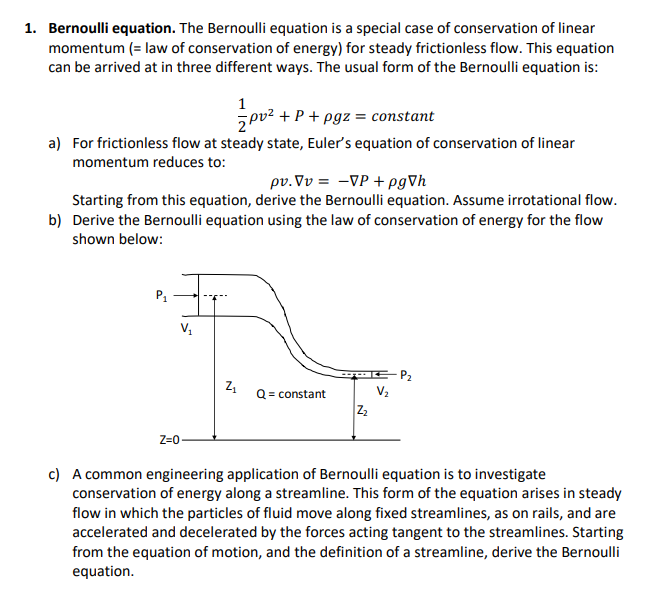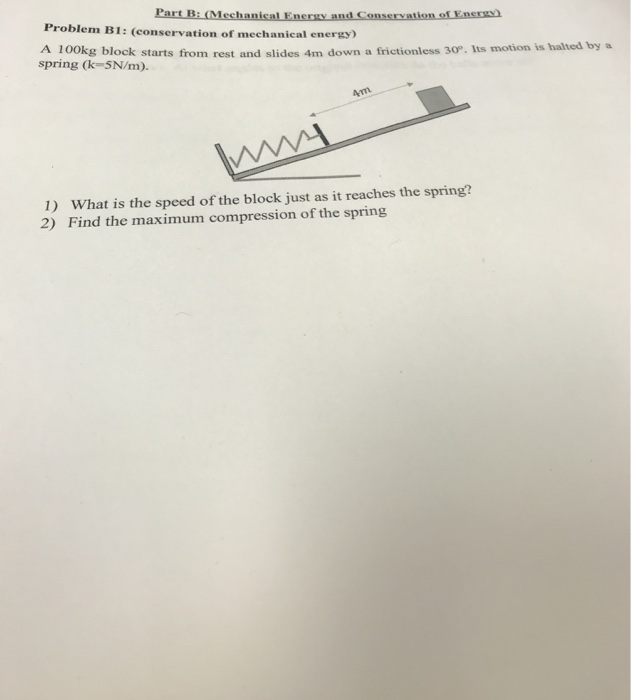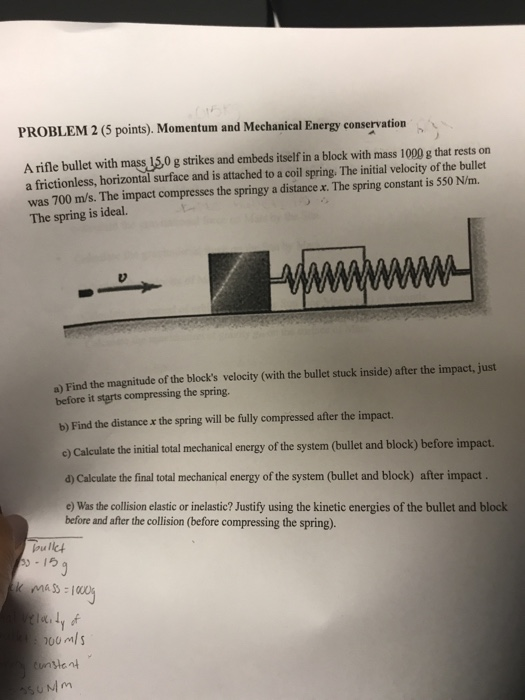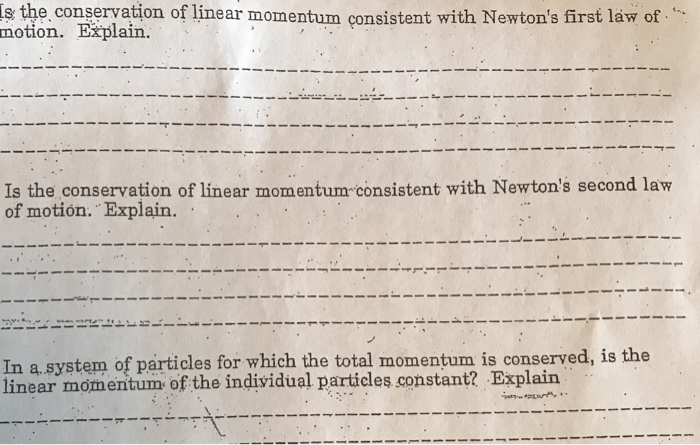Question

# State the laws of conservation of linear momentum and mechanical energy.

State the laws of conservation of linear momentum and mechanical energy.

The law of conservation of linear momentum states that if no external forces act on the system of two colliding objects, then the vector sum of the linear momentum of each body remains constant and is not affected by their mutual interaction.

The law of conservation of energy is an empirical law of physics. It states that the total amount of energy in an isolated system remains constant over time (is said to be conserved over time). A consequence of this law is that energy can neither be created nor destroyed: it can only be transformed from one state to another.

conservation of linear momentum -

A conservation law stating that the total linear momentum of a closed system remains constant through time, regardless of other possible changes within the system

conservation of  mechanical energy -

According to the law of conservation of mechanical energy, the mechanical energy of an isolated system remains constant in time, as long as the system is free of friction and other non-conservative forces

The law of conservation of linear momentum states that if no external forces act on the system of two colliding objects, then the vector sum of the linear momentum of each body remains constant and is not affected by their mutual interaction.

According to the law of conservation of mechanical energy, the mechanical energy of an isolated system remains constant in time, as long as the system is free of friction and other non-conservative forces.

the linear momentum of a system remains constant when it is not acted upon by an external force

the energy of the system is constant,, i.e. T E = PE + KE =constant ( ignoring frictinal, heat and sound losses)

The law of conservation of linear momentum states that if no external forces act on the system of two colliding objects, then the vector sum of the linear momentum of each body remains constant and is not affected by their mutual interaction.

According to the law of conservation of mechanical energy, the mechanical energy of an isolated system remains constant in time, as long as the system is free of friction and other non-conservative forces. In any real situation, frictional forces and other non-conservative forces are present, but in many cases their effects on the system are so small that the law of conservation of mechanical energy can be used as a fairapproximation. Though energy cannot be created or destroyed in an isolated system, it can be converted to another form of energy.

#### Earn Coins

Coins can be redeemed for fabulous gifts.

Similar Homework Help Questions
• ### Show that the laws of momentum and energy conservation forbid the complete absorption of a photon...

Show that the laws of momentum and energy conservation forbid the complete absorption of a photon by a free electron. (Note: This is NOT the photoelectric effect. In the photoelectric effect, the electron is not free; the metal participates in momentum and energy conservation.

• ### Conservation of Linear MomentumConservation of Linear MomentumUsing the simulation (http://www3.interscience.wiley.com:8100/legacy/college/halliday/0471320005/simulations6e/)ChooseConservation of Linear Momentumthe answer the following questions. (a) When the simulation is run a constant force directed right is applied to ball 1 (the ball on the left) for 0.2 seconds. CHOOSE ALL THE CORRECT STATEMENTS about this situation from the list below. Note that "the total mass of the system" is defined as the sum of mass 1 and mass 2, and "the total momentum of the system" is the same as...

• ### Bernoulli equation. The Bernoulli equation is a special case of conservation of linear momentum law of...Bernoulli equation. The Bernoulli equation is a special case of conservation of linear momentum law of conservation of energy) for steady frictionless flow. This equation can be arrived at in three different ways. The usual form of the Bernoulli equation is: 1. pv2 + P + ?9z-constant a) For frictionless flow at steady state, Euler's equation of conservation of linear momentum reduces to: Starting from this equation, derive the Bernoulli equation. Assume irrotational flow. Derive the Bernoulli equation using the...

• ### Part B: (Mechanical Energy and Conservation of Energy Problem B1: (conservation of mechanical energy) KS block...Part B: (Mechanical Energy and Conservation of Energy Problem B1: (conservation of mechanical energy) KS block starts from rest and slides 4m down a frictionless 30°. Its motion is halted by a spring (k=5N/m). ww 1) What is the speed of the block just as it reaches the spring? 2) Find the maximum compression of the spring

• ### 1) Under what circumstances is mechanical energy conserved? Under what circumstances is momentum conserved? How is...

1) Under what circumstances is mechanical energy conserved? Under what circumstances is momentum conserved? How is this different than the criteria for conservation of mechanical energy?

• ### PROBLEM 2 (5 points). Momentum and Mechanical Energy conservation A rifle bullet with mass 150 g...PROBLEM 2 (5 points). Momentum and Mechanical Energy conservation A rifle bullet with mass 150 g strikes and embeds itself in a block with mass 1000 g that rests on a frictionless, horizontal surface and is attached to a coil spring. The initial velocity of the bullet was 700 m/s. The impact compresses the springy a distance x. The spring constant is 550 N/m. The spring is ideal. a) Find the magnitude of the block's velocity (with the bullet stuck...

• ### s the conservation of linear momentum consistent with Newton's first láw of. motion. Explain. Is the...s the conservation of linear momentum consistent with Newton's first láw of. motion. Explain. Is the conservation of linear momentum-consistent with Newton's second law of motión. Explain. In a.system of particles for which the total momentum is conserved, is the linear momentum of the individual particles constant? Explain

• ### Use conservation of energy and momentum to find the kinetic energy of the recoiling nucleus in...

A 57Fe nucleus at rest emits a 14.0-keV photon. Use conservation of energy and momentum to find the kinetic energy of the recoiling nucleus in electron volts. Use Mc2= 8.60 10-9 J for the final state of the 57Fe nucleus.

• ### State the Law of Conservation of Momentum? Give an example of a situation where momentum is...

State the Law of Conservation of Momentum? Give an example of a situation where momentum is conserved.

• ### Momentum Theory Use one-dimensional conservation of momentum together with conservation of mass (continuity) and energy (Bernoulli’s...

Momentum Theory Use one-dimensional conservation of momentum together with conservation of mass (continuity) and energy (Bernoulli’s equation = mechanical energy) to derive the power an ideal, frictionless wind turbine with an infinite number of blades, uniform thrust over the rotor area and a non-rotating wake can extract from the wind. Formulate the derivation in terms of the fractional decrease in wind velocity between the velocity far upstream and at the turbine rotor, ? = (? − ?)/?, also called “axial...